Area of Path and Cross roads - Worksheet

Chapter 9 Class 7 Perimeter and Area
Serial order wise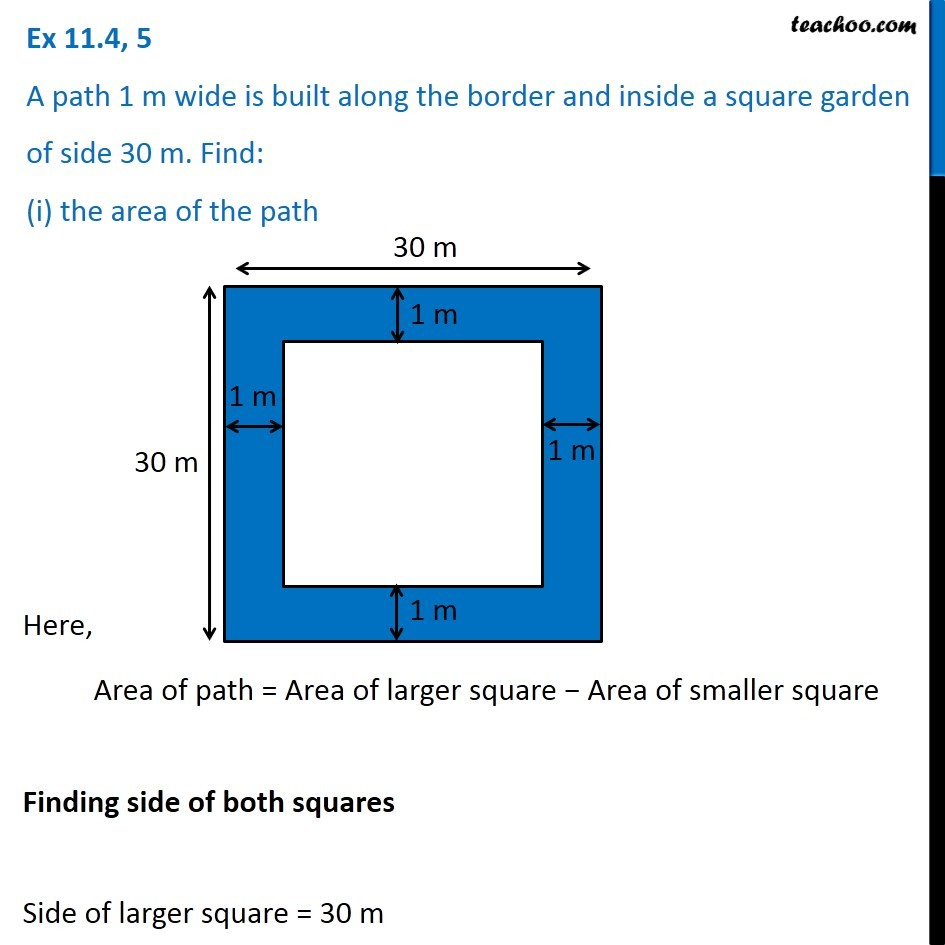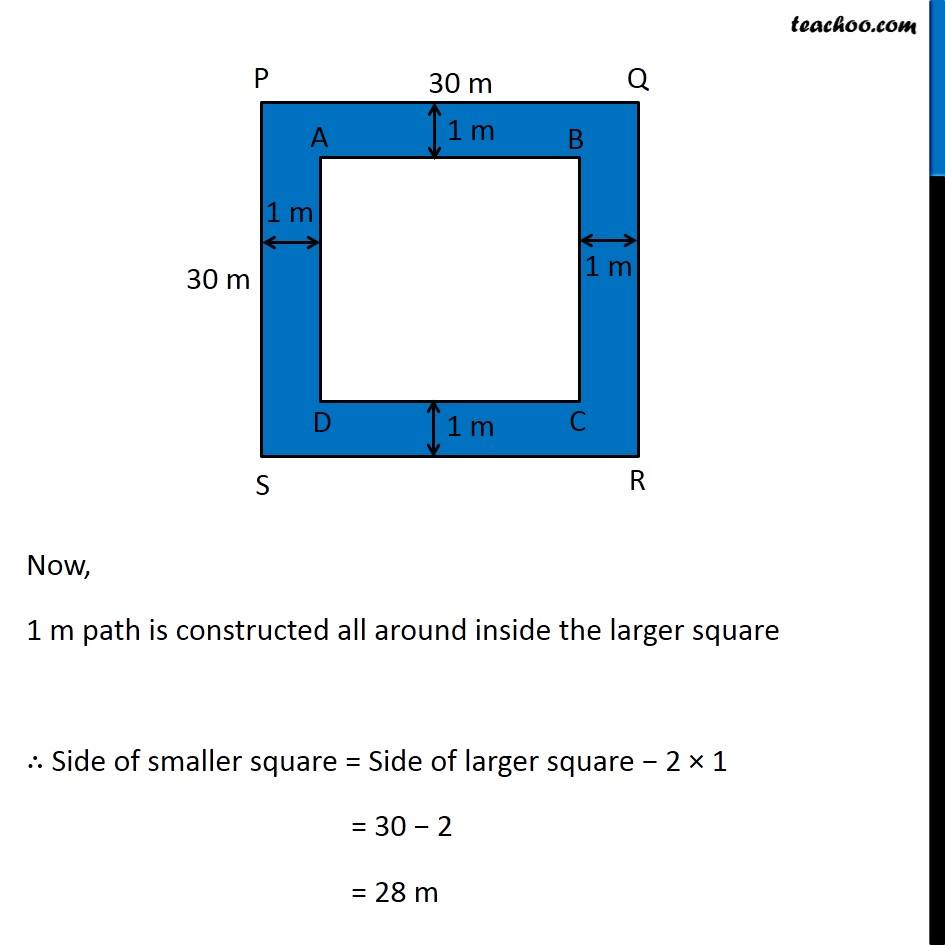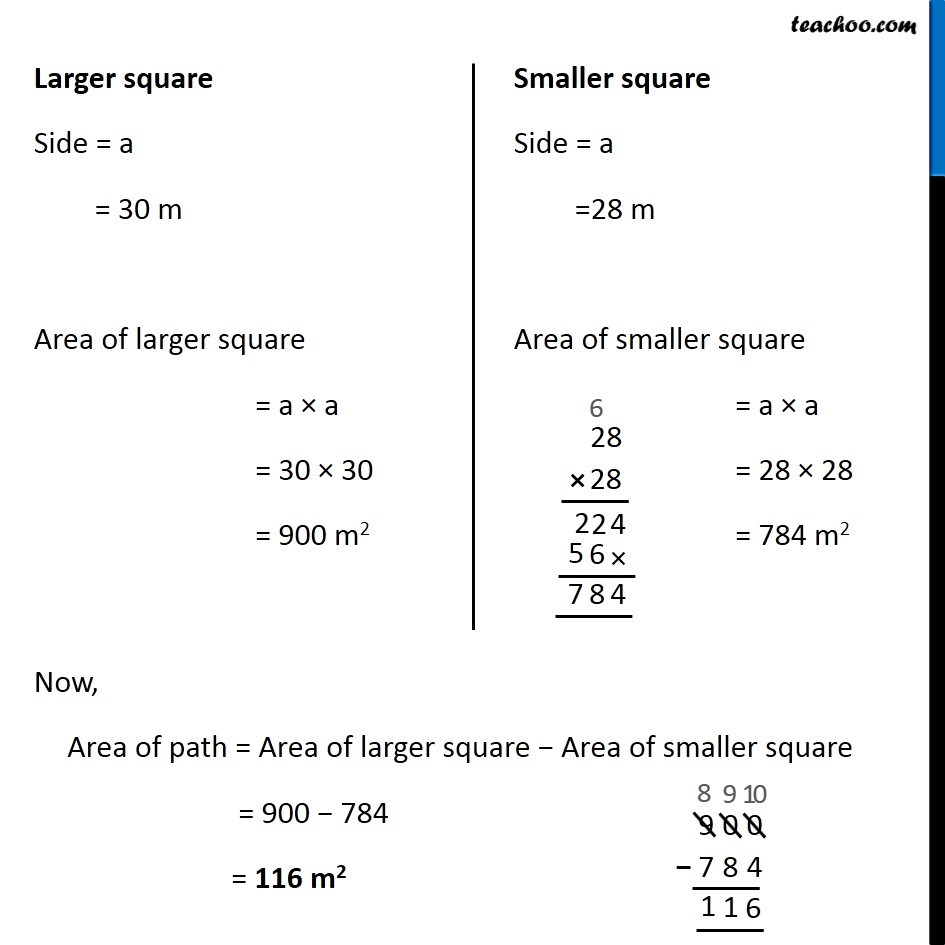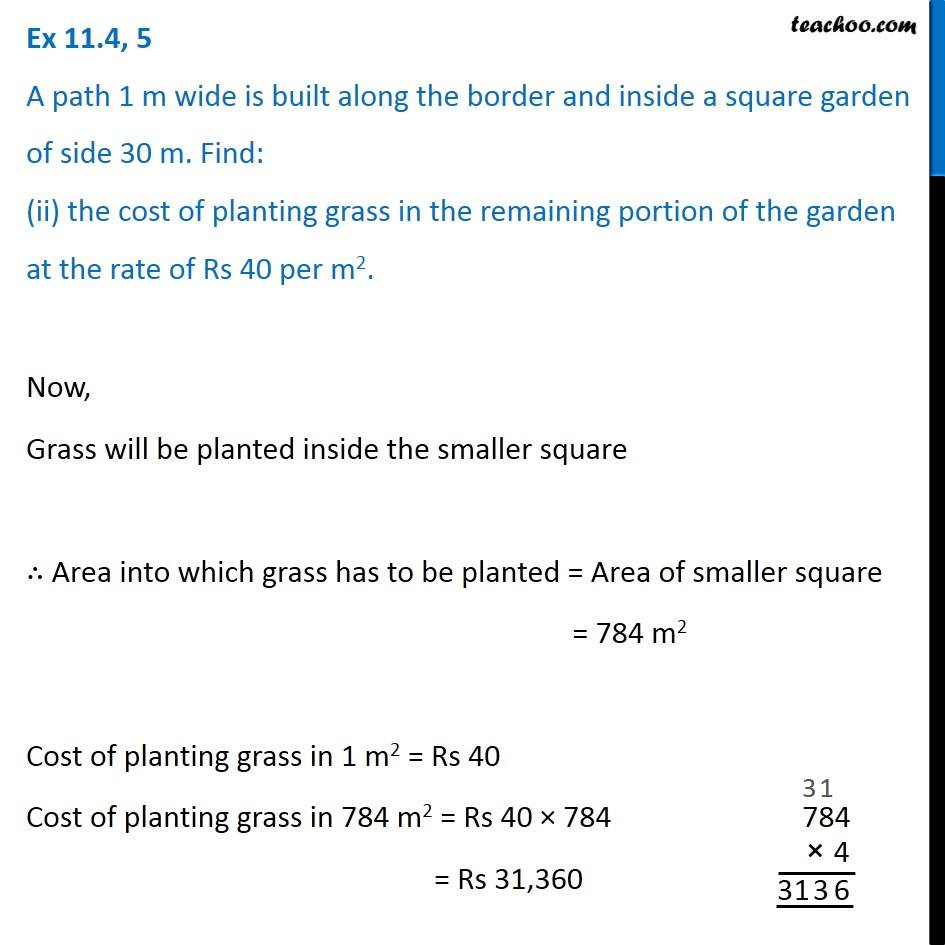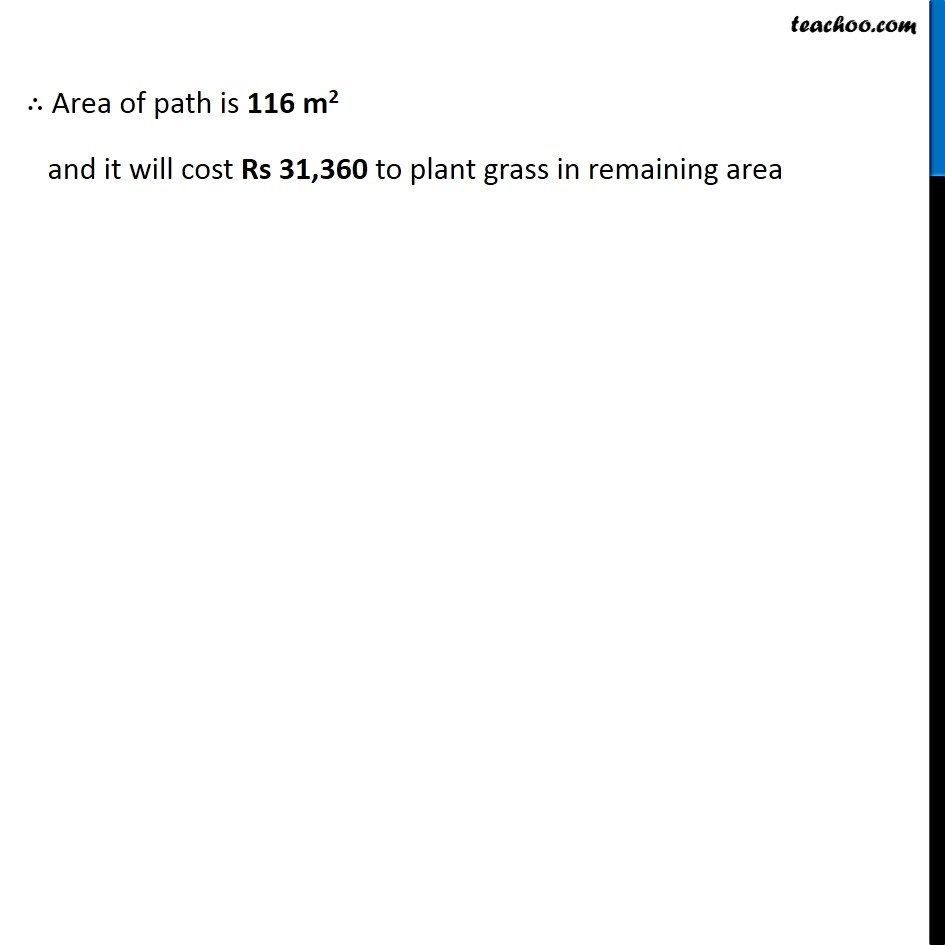Learn in your speed, with individual attention - Teachoo Maths 1-on-1 Class

### Transcript

Question 5 A path 1 m wide is built along the border and inside a square garden of side 30 m. Find: (i) the area of the path Here, Area of path = Area of larger square − Area of smaller square Finding side of both squares Side of larger square = 30 m Now, 1 m path is constructed all around inside the larger square ∴ Side of smaller square = Side of larger square − 2 × 1 = 30 − 2 = 28 m Larger square Side = a = 30 m Area of larger square = a × a = 30 × 30 = 900 m2 Smaller square Side = a =28 m Area of smaller square = a × a = 28 × 28 = 784 m2 Now, Area of path = Area of larger square − Area of smaller square = 900 − 784 = 116 m2 Question 5 A path 1 m wide is built along the border and inside a square garden of side 30 m. Find: (ii) the cost of planting grass in the remaining portion of the garden at the rate of Rs 40 per m2. Now, Grass will be planted inside the smaller square ∴ Area into which grass has to be planted = Area of smaller square = 784 m2 Cost of planting grass in 1 m2 = Rs 40 Cost of planting grass in 784 m2 = Rs 40 × 784 = Rs 31,360 ∴ Area of path is 116 m2 and it will cost Rs 31,360 to plant grass in remaining area